## Friday, August 28, 2009

### hypocycloid scramblerThe scrambler amusement park ride gives a visceral sense for how circular motions can be added together to create a trajectory that scarecely resembles a circle itself. If you watch (or get on) the ride, you'll see (or feel) that while the ride consists of a circular structure spinning on a larger spinning circle, riders seem to move in triangular or square paths (it was moving too fast for me to tell which).
When I tried to model the scrambler in Geometer's Sketchpad to learn more about the path that its riders follow, I chose to have point A traveling in one direction on a large circle, while point B travelled in the opposite direction on a circle whose radius was half the size of the first, centered on A. Both points were set to travel at the same speed. This gave a path very much like the scrambler's - a curved triangle.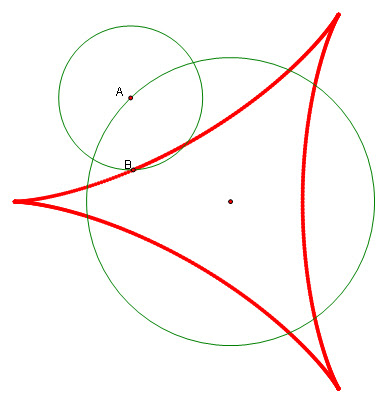Different choices for the relative sizes and speeds of the circles and points give different curves. In this case, having the circles move in opposite directions creates a curve that is the simplest hypocycloid, while having the circles move in the same direction creates the simplest epicycloid.Hypocycloids are usually defined by having the small circle roll along the inside of the larger circle, while epicycloids are defined by having the small circle roll along the outside. By choosing the right rotation speed and sizes, you can get the same curves by having the small circle centered on the circumferences of the large circle, as I did when modeling the scrambler.
There are lots of ways to explore this model further, but one that appealed to me was to try to add additional circles spinning on top of the existing ones, with each additional circle having half the radius of the one it sits on. Having the circles spin in different directions generates very different curves (a further restriction was to start all circular motion in phase with all the points initially lined up). By varying the spin directions, two circles generated two paths (the epicycloid and hypocycloid above), while three circles generated four paths, shown below.One great thing about GSP is that you can look at these curves synthetically, where you build them up out of points, lines, and circles, or analytically, where you provide explicit equations for the coordinates of the points that lie on the curves. It turns out that for the family of curves built out of these circles, each having half the radius of the preceding circle, the equations are:

Where we are letting the big circle have radius = 1, n is the number of circles that have been added to the initial base circle, and the coefficients in the expression for y are chosen to be positive or negative to yield different curves (a positive coefficient on the i-th term causes the i-th circle to move counterclockwise, and a negative coefficient causes it to move clockwise). When we choose all positive coefficients and look at n from 0 to 4, we get an interesting evolution of the epicycloid: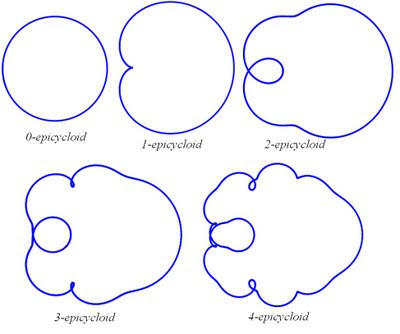If you allow negative coefficients in the y expression and allow allow the circles to spin in different directions, you'll find that the '4th generation' of this family includes some very oddly shaped curves:

The GSP file used to create up to the 2nd generation of these curves is here.

Update: The first few generations of these curves are easy to make using Desmos.

Scrambler fractal
Brain curve

## Wednesday, August 19, 2009

### origamiExploring the mathematics of origami has been a pursuit of recreational mathematicians, educators, and mathematical researchers for some time. Martin Gardner's chapter on the mathematics of origami in The Second Book of Mathematical Puzzles and Diversions remains a good starting point for anyone who is curious. Barry Cipra's article in the October 2001 SIAM News is more recent survey that stresses the applications of the mathematical approach to origami (for more applications see Simon Guest on 'deployable structures').

Three mathematicians that are at the forefront of the research end of this topic are Robert Lang, Erik Demaine, and Tom Hull. All three are featured in the Institute for Figuring's online exhibit "Mathematical Paper Folding." Erik Demaine has a downloadable book chapter that provides a nice overview of recent advances in the subject. Robert Lang's paper with Roger C. Alperin outlines some of the axiomatic approaches to the mathematics of origami. There are some down loadable worksheets from Tom Hull's book, Project Origami, here.

One set of activities in Hull's book introduces Kazuo Haga's "origamics," which is an approach to teaching the mathematics of origami that emphasizes experimentation and discovery. One of explorations asks students to look at making a single fold and observing what polygon is created by the "turned up part" (do you get a triangle, square, or other polygon? when and why do different polygons occur?). The appeal of this activity is its simplicity, a simplicity that also allows it to be modeled effectively in Geometer's Sketchpad (a GSP file for this activity is here, and solutions here).

In addition to the activities in this book (and many others), there are some great videos available that show how origami can be integrated into mathematics education. A webinar for Ontario teachers is archived here, and an inspiring overview of an Israeli program ('Origametria') is shown here. Using origami in education is not new - in the West it was introduced by Friedrich Fröbel along with the concept of kindergarten (see another TIFF online exhibit, Inventing Kindergarten). The history of teaching with origami in Japan, of course, goes back further still.

Despite its history, this is definitely a very current subject: the documentary film Between the Folds is on the film festival and conference circuit right now, and is apparently going to be generally available for purchase in the fall.

Beautiful mathematics is only part of the appeal of origami - the stunning transformations that can be achieved through origami are what really attract people to it - witness Sara Adam's miraculous construction of the Robert Lang cuckoo clock.

## Friday, August 7, 2009

### triangulo-triangular numbers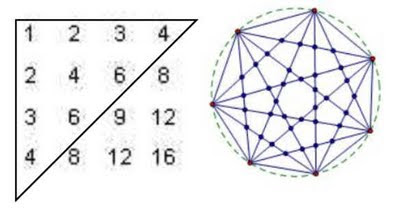I recently learned from Janet Beery's paper, Formulating Figurate Numbers, that mathematicians have been referring to the 4-dimensional triangular numbers as the 'triangulo-triangular' numbers for about 400 years. Here are the 'triangular' numbers of dimension 0 through 5:These are built by summing (or stacking, if you like the visual analogy) triangular numbers of one dimension less:I've stumbled across the triangulo-triangulars a few times recently - here are two places where you see them.

1. upper triangle sums in multiplication tables

Take a standard school multiplication table and split it along the diagonal that goes from bottom left to upper right. If you sum up all the numbers from the left hand side (including the middle diagonal), you get a triangulo-triangular number.You can work out why this happens using ideas in this post.

2. connecting n+3 points on a circle

If you place n+3 points on a circle and connect them all, then the maximum number of line crossings in the circle is the nth triangulo-triangular number.The triangulo-triangulars come up in this context because to get a crossing you have to choose four of the n+3 points on the circle (drawing lines between the four chosen points gives you your intersection point), and "n+3 choose 4" gives you the triangulo-triangulars. You can see this also in how they occur in the r=4 column of Pascal's Triangle:Just as they show up in several contexts, there are several ways to express a formula for the triangulo-triangulars. The direct formula below shows their quartic nature:and the direct formula comes easily from the combinatorial one: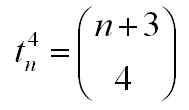There is also a nice ordinary generating function for them, as described here.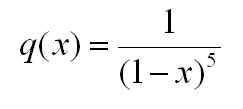## Wednesday, August 5, 2009

### ~ 95 mathematiciansThe BBC4 documentary Dangerous Knowledge (on youtube and on watchmath), although worth watching, promotes the popular but unfortunate notion that mathematicians are prone to madness. Timothy Gowers recently noted the tendency to portray mathematicians as outcasts on the brink of madness, and a quick survey of films that mention math will likely bear his observations out (in keeping with this trend, I am waiting for a film of the life of Grothendieck).

When mathematicians write about themselves, they don't tend to mention madness as an occupational hazard. The role played by social and mental dysfunction seems to be one of the key differences that emerge when we compare what outsiders think about mathematicians and what mathematicians think about themselves.

I've been collecting links to the homepages of mathematicians, and have about 95 here. Many pages have links to preprints and other resources. Generally they don't seem to be unstable, but you never know.

The image at the top of the post was taken from Phrenology, or, The doctrine of the mind (1825), by Johann Gaspar Spurzheim, and purports to show the location of the calculation organ - which may be dangerously close to organs that govern mental equilibrium.